Perple_X Equilibrium Reactive Transport Calculations: FRAC2D Model Configuration

Contents

Files for the FRAC2D example

Figures:

Introduction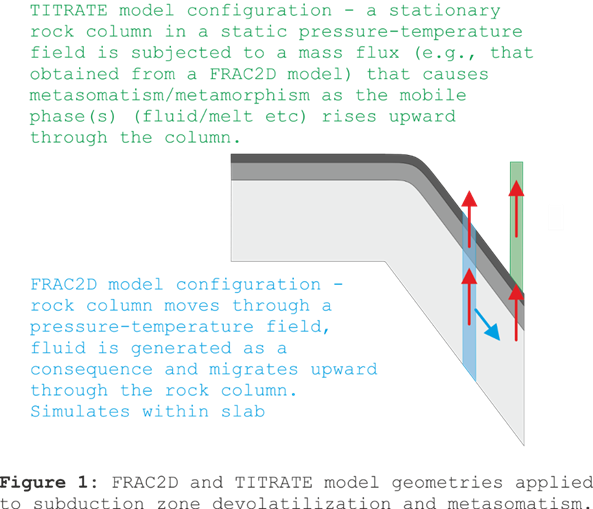Perple_X is configured to do two general types of equilibrium reactive transport models, FRAC2D and TITRATE (Figure 1). Both configurations are referred to within Perple_X as 2d-phase fractionation models (Connolly 2005). In these calculations a 1-dimensional column of rock is subject to perturbations that lead to the formation of one or more mobile phases (e.g., melt and low-density electrolytic or molecular fluids). The rate at which the perturbations are applied adds a second effective dimension, time, to the model. The mobile phase(s), i.e., fractionated phase, moves upward through the column until it is consumed or passes through the top of the column. The two types of calculations are distinguished by the mechanism for perturbing the initial equilibrium condition: in FRAC2D calculations, the perturbation is caused by changing pressure-temperature conditions, as occurs within a subducted slab or during regional metamorphism; in TITRATE calculations the perturbation is a mass flux (e.g., the presumed composition of a mobile phase) to the base of the column, as occurs in the mantle wedge during subduction and other metasomatic processes. This page documents the FRAC2D configuration, refer to the TITRATE page for an outline of TITRATE configuration. FRAC2D and TITRATE represent limiting cases, the intermediate case in which the column moves through a dynamic P-T field and is simultaneously subject to a basal mass flux can be computed as a variant of the FRAC2D configuration in which the TITRATE variable (see below for definition of all input/output variables) is set.

The input files for the FRAC2D example outlined here are at www.perplex.ethz.ch/perplex/examples/FRAC2D are designated by the project name frac2d. The fractionated, or mobile phase, is represented by the solution model COH-Fluid+, a model for low-density C-O-H-S fluids. Depending on the aq_lagged_speciation option specified in the Perple_X option file, COH-Fluid+ may represent either molecular or electrolytic fluids (Connolly and Galvez, 2018). The example here uses the molecular version of the model and thus corresponds to an up-date of the subduction model used by Connolly (2005). It is advisable to work with the molecular model before attempting the electrolytic model which introduces additional complexity and uncertainty. As in all Perple_X calculations, the project name has no special significance, the name frac2d used here is chosen solely for purpose of the example. In this example, equilibrium phase relations are computed for a column of rock representing the lithologies of the upper 24.5 km of an oceanic slab as it is subducted incrementally from 80 to 200 km depth, the column is oriented vertically because it is assumed this is the direction of fluid flow. Consequently, the height of the column is 24.5 km / cosine (45 degrees) ~ 34.6 km (see Fig 6 of Connolly 2005), where 45 degrees is the model slab dip. For purposes of the calculation, the column is discretized as a set of representative nodes. After each depth increment, beginning from the lowest representative node, equilibrium phase relations are computed. If the mobile phase is stable, its mass is removed (fractionated) from the node and added to the overlying node. The procedure is then repeated for the overlying node until, ultimately, the top of the column has been reached. This model carries strong assumptions about the nature of fluid generation and flow. Specifically, these assumptions are that fluid generation proceeds from the base of the column upwards, that fluid flow is instantaneous on the subduction time scale (DT0) and that the flow is pervasive and vertical. There is little basis for the first assumption, although the case can be made that any fluid accumulation at depth will induce hydro-mechanical instabilities that causes the fluid at depth to catch-up fluid at shallower depths (Connolly 1997). Experiments with alternative assumptions, e.g., that fluid generation proceeds from the top downward, show that this assumption does not have profound consequences for mass transport. Pervasive flow maximizes the efficacy of mass transport through the slab and is therefore a useful extremal scenario. The alternative extremal scenario, that fluid flow is perfectly channelized by fractures or tube-like porosity waves (Connolly & Podladchikov, 2007, 2013; Connolly 2010) corresponds to simple Rayleigh fractionation (e.g., Section 4.4 of Connolly & Galvez 2018) and is not considered further here. The independent variables for the calculation the depth of the slab/mantle interface (Z0) and the vertical height above the base of the slab column (DZ), the pressure-temperature field from a geodynamic model is expressed as a function of Z0 and DZ (see below, also Fig 7 of Connolly 2005). The model neglects the small effect of the divergence in the mass flux on density and, for the purposes of relating pressure and depth, assumes a constant density throughout the column and overlying rocks.

The primary input file for the calculation, frac2d.dat, is generated using BUILD, by choosing computational mode 7 (2-d Phase fractionation). In contrast to most Perple_X calculations in the case of FRAC2D calculations, this input file specifies only the data sources, thermodynamic components, and solution models to be considered during the calculation. A second auxiliary file, frac2d.aux, specifies the column lithologies, the model geometry, and the data necessary to define the pressure-temperature field during subduction. The auxiliary input file is generated manually, the primary purpose of this document is to explain the data in the frac2d.aux example so that it can be used as a template for new calculations.

frac2d_noaq.dat                 - the primary input file (Figure 3).

frac2d_noaq.aux                 - the auxiliary input file (Figure 3).

frac2d_noaq_perplex_option.dat  - the run time option file (Figure 3).

frac2d_aq.dat                   - variant with lagged-aqueous speciation enabled (Figures 2 and 4), the primary input file.

frac2d_aq.aux                   - variant with lagged-aqueous speciation enabled (Figures 2 and 4), the auxiliary input file.

frac2d_aq_perplex_option.dat    - variant with lagged-aqueous speciation enabled (Figures 2 and 4), the run time option file.

solution_model.dat              - the solution model file.

DEW13HP622ver_elements.dat      - the thermodynamic data file.

NOTES: 1) The DEW13 aqueous solute data-base was not converted to Perple_X format using the default options specified in the DEW spreadsheet, it is used here only for purposes of demonstration, the DEW17 aqueous solute data-base should be used for quantitative applications. 2) These files can only be read by 6.8.6+ versions of Perple_X.

A) Run BUILD to create the primary input file, e.g., frac2d.dat.

B) Create the auxiliary input file, e.g., frac2d.aux.

C) Run VERTEX, answer yes to the fractionate phases prompt and fractionate the mobile phases of interest (e.g., COH-Fluid+).

D) Plot and/or analyze phase relations using PSSECT and WERAMI (see section 3, Figures 2, 3, 4). Note that in fractionation calculations, the data reported by VERTEX include the amount and composition of the mobile phase prior to fractionation. In FRAC2D calculations, the amount of the mobile present at a given height within the column is the product of the mobile phase generated at that height plus any mass gained by fractionation of the mobile phase at greater depths.

E) If you are unfamiliar with Perple_X, familiarize yourself with the programs by following a tutorial such as www.perplex.ethz.ch/perplex_66_seismic_velocity.html.

F) Set auto_refine to off or manual, using the low-resolution (exploratory stage) result will identify problems quickly, these problems must be resolved before a high-resolution (auto-refine stage) result is attempted.

G) Before configuring your own calculation, begin at step C, above, using the input files at www.perplex.ethz.ch/perplex/examples/frac2d. Verify that you are able to obtain, understand, and analyze the output generated by the example.

H) Increase complexity gradually: Do not begin with an electrolytic multi-component fluid, rather begin with water, add molecular volatiles, and lastly add solutes. Do not begin with complex lithologic chemistry, rather begin with elements in a single oxidation state and exclude minor and/or poorly constrained elements. Construct phase diagram sections for each lithology within your model rock column over the entire pressure-temperature range of interest. If the phase relations in these sections do not make sense, then the phase relations in a FRAC2D calculation certainly will not make sense.

Explanation/Definition of FRAC2D Variables

Variables used to describe the FRAC2D calculation, unless otherwise indicated these are specified in the frac2d.aux file or computed on output:

AREA    - the cross-sectional area of the subducted rock column, AREA = MASS/ZBOX/RHO.

ANNEAL  - if .true., then at the initial condition of the calculation: a) if fluid is stable at any node in the column, it is removed and the composition of the node adjusted accordingly; b) the representative masses (MASS) of all nodes are renormalized to 1 kg.

C       - the number of thermodynamic components specified in frac2d.dat

CLAY_1_1 ... CLAY_1_C

...      ... ...

CLAY_N_1 ... CLAY_N_C - the molar compositions of the N column lithologies, from the base of the column upward. The components in the composition must be entered in the same order as the components are entered in frac2d.dat. For calculations in which the fluid is electrolytic it is advisable to use elemental components. See www.perplex.ethz.ch/perplex/faq/oxide_to_elemental_component conversion.pdf for an illustration of the oxide-to-elemental conversion used for the frac2d example. Note that these compositions are extensive. If ANNEAL is .true., the mass of the composition will be automatically adjusted to 1 kg. If ANNEAL is .false., the molar mass of each layer composition should be comparable.

C_1_0    ... C_1_NORD

...      ... ...

C_NPOLY_0... C_NPOLY_NORD - the coefficients of the NPOLY NORD'th order polynomials that define the within slab geotherms, such that T(K) on the N'th geotherm is T_N = sum(C_N_I*ZI, I = 0,...,NORD). NOTE: Perple_X does (cannot) not test that the polynomials are valid, therefore it is advisable to plot the geotherms over the entire depth range relevant to a calculation. In some cases it may be impossible to represent a geotherm with a single polynomial, when this is the case use the PZFUNC option to specify the geotherms or explicit P(Z0,DZ)-T(Z0,DZ) functions.

DIP     - slab dip in degrees

DPDZ    - the pressure gradient with depth (bar/m). DPDZ = RHO(kg/m3)*g(m2/s)*(1 bar/105 Pascal), where g is gravitational acceleration (10 m/s2) and RHO is the average density of the column and any overlying rock. Perple_X can be modified to compute a thermodynamically consistent pressure for a loaded self-gravitating column; such complications seem unwarranted in light of other sources of uncertainty.

DT0     - the time interval (s) corresponding to one depth increment of the column, DT0 = DZ0/cosine(DIP)/V_SUB

DYNAMIC - if .true. Pressure and temperature are a function of the Z0-DZ coordinate. If DYNAMIC is .false., pressure and temperature are solely a function of DZ.

DZ      - height above the base of the column; as DZ = 0 at Z0, DZ is negative within the column (see ZBOX).

DZ0     - the incremental change in depth of the column during subduction, DZ0 = (Z0MIN-Z0MAX)/NINT

DZGEO_1...DZGEO_NPOLY - the within slab (orthogonal) depths of the NPOLY geotherms that define the within slab P-T field.

MASS    - the mass (g) associated with a representative node within the column. If ANNEAL is true, MASS is automatically adjusted to 1 kg; otherwise MASS is the mass corresponding to the extensive molar composition of the layer composition (CLAY_I_J, J = 1...C).

MASS_I  - the mass (g) of a component transported by mobile phase I (e.g., fluid and/or melt).

NINT    - the number of depth increments as the column is subducted from Z0MIN to Z0MAX. NINT = 1d_path_nodes - 1, where 1d_path_nodes is specified in frac2d_perplex_option.dat.

NORD    - the order of the polynomials used to describe the within slab P-T field.

NPOLY   - the number of geotherms used to describe the within slab P-T field, three should be adequate for most purposes. The within column temperature is computed by fitting an (NPOLY-1)'th order polynomial to the geotherms (see Connolly 2005).

RHO     - the average density (kg/m3) of the column and overlying rock (see DPDZ), RHO = DPDZ/g*(105 Pa/bar)

P       - pressure (bar) at any point within the column, P = Z*DPDZ.

Q       - the flux (g/m2-s) of a component transported by mobile phase I through the column over time interval DT0 (e.g., fluid or melt) Q = MASS_I/AREA/DT0 (see step D below).

QINT    - the time-integrated flux (g/m2) transported through the column by mobile phase I (see step D below).

PZFUNC  - if .true., then internal functions are used to compute the P-T field of the column, e.g., from analytical functions (Davies, GJI, 1999) or tabulated results from, e.g., a geodynamic model. This option requires that the user program the functions.

T       - temperature (K).

TITRATE - if .true., then the column is subject to a basal mass flux.

VERBOS  - if .true., then VERTEX prints the amount and composition of the mobile phase at each node to the console. Additionally, if ANNEAL is also .true., then VERTEX outputs the initial composition at each node and the average composition of each layer after annealing.

V_SUB   - the subduction rate (m/s).

V_HOR   - the horizontal component of the slab velocity (m/s), V_HOR = V_SUB*cosine(DIP).

Z       - the absolute depth (m) of any point within the column, as DZ increases upward, Z = Z0-DZ.

ZBOX    - the vertical unit of discretization for the column in meters, i.e., the rock column is composed of boxes with a vertical dimension of ZBOX. The representative nodes of the column are located at DZ_I = -ZTOT+ZBOX*(1/2+[I-1]); thus the representative nodes at the top and bottom of the column are at DZ_TOP = -ZBOX/2 and DZ_BOT = -ZTOT+ZBOX/2, respectively. NOTE: the nodal coordinates of a layer should be entered in WERAMI or PSSECT to compute fluxes, e.g., to compute the flux across the top of the column, compute properties at column depth -ZBOX/2.

ZLAY_1...ZLAY_N - the vertical thicknesses (m) of the N lithological layers within the column, numbered from the base of the column upward. The vertical thickness is equal to the thickness of the layer orthogonal to the slab interface divided by the Cosine of the slab dip (DIP). NOTE that the layers are discretized with a resolution ZBOX, thus the layer thicknesses should be an integer multiple of ZBOX. If this is not true, then the model layer thickness will be (ZLAY_I\ZBOX)*ZBOX, where \ denotes the integer quotient. When a computation is run in VERTEX, the actual layer thicknesses and the nodal coordinates of the top and bottom of each layer are output to the console.

ZSURF   - value of the depth coordinate at the surface (m), used for the computation of pressure at depth, i.e., P(bar) = (Z0-DZ)*DPDZ.

ZTOT    - the total vertical thickness of column (m), ZTOT = sum(ZLAY_I, I = 1...N).

Z0      - depth (m) at the top of the column (m), i.e., the depth of the slab/wedge interface.

Z0MIN   - the initial depth (m) of the slab/mantle interface

Z0MAX   - the final depth (m) of the slab/mantle interface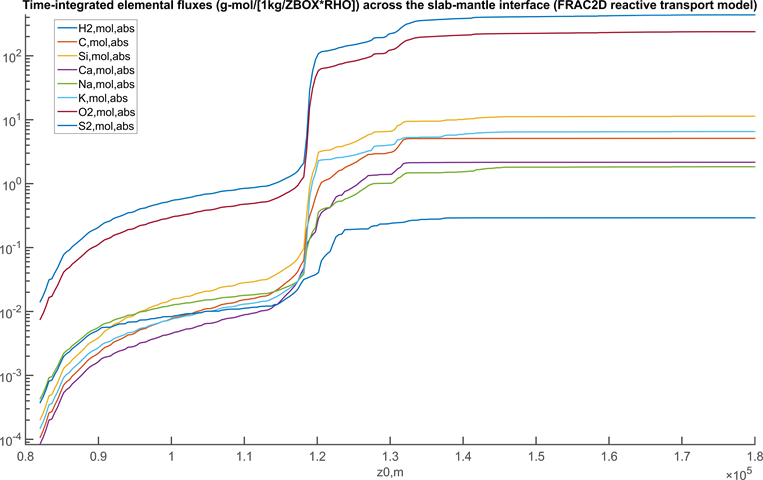Figure 2: Time-integrated elemental fluxes across the slab-mantle interface. The negligible fluxes of Fe, Al, and Mg are not shown. NOTES: 1) the fluxes must be scaled by ZBOX and RHO (see variable definitions above) to obtain rational units (e.g., g-mol/m2); 2) the option file linked from this page disables electrolytic fluid speciation so that carbon, sulfur, hydrogen, and oxygen are the only mobile elements. To reproduce the figure presented here the aq_lagged_speciation option must be set to T (true).

Computation and Plotting of Mass Fluxes

A) Set the absolute option to T in frac2d_perplex_option.dat.

B) Set the cumulative option to T in frac2d_perplex_option.dat if cumulative fluxes are desired, otherwise the fluxes are instantaneous, i.e., the average flux over the time interval DT0.

C) After setting the above options, start WERAMI:

i) Select operation mode 3 (properties a long a 1d path).

ii) Construct a linear profile, from (Z0 = Z0MIN, DZ = -ZBOX/2) to (Z0 = Z0MAX, DZ = -ZBOX/2).

iii) For optimal resolution specify NINT + 1 points for the profile.

iv) Select property 36 (all phase and/or system properties).

v) Specify option 2 (properties of a phase).

vi) Specify the name of the fractionated phase, e.g., COH-Fluid+.

vii) Answer y or n to the Include fluid in ... properties prompt, the answer is irrelevant for present purposes.

iix) The output is in the indicated tab file, e.g., frac2d_1.tab.

ix) Exit.

D) Plot the amount of the component of interest (e.g., S2_g) using perple_x_simple_plot (MATLAB), PSTABLE, or PYWERAMI. If the cumulative option is F or default, the plotted quantity corresponds to the MASS_I (g) of the component that is transported by the fluid across the slab/mantle interface (at DZ+ZBOX/2 = 0) as the top of the column is subducted from depth Z0 to Z0+DZ0. The instantaneous flux, i.e., the flux averaged over the time interval DT0, Q (g/m2-s) = MASS_I/AREA/DT0. If the cumulative option is T, then the plotted quantity is the cumulative mass TOT_MASS_I of the component transported by the mobile phase, the time-integrated mass flux QINT (g/m2) is TOT_MASS_I/AREA. The data in the tab file can be edited in a spreadsheet program so that Q and/or QINT are output directly. Alternatively, the axes of the plot may be rescaled to indicate the appropriate flux.

E) If a calculation is done with more than one mobile phase (e.g., melt and low-density fluid), step C must be repeated for each phase and the results summed to obtain the total mass flux transported by the mobile phases.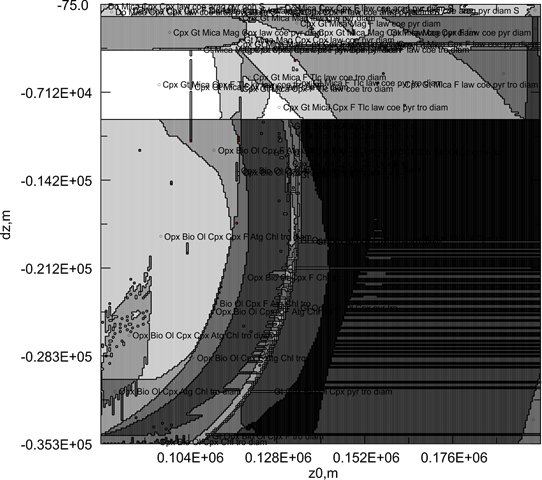Figure 3: Phase relations within the subducted column as a function of column depth (Z0) and height within the column (DZ) plotted with PSSECT. Irregularities occur because small oscillations in the bulk composition at the representative nodes caused by metasomatism affect the stability of minor phases. These oscillations can be dampened by increasing NINT and decreasing ZBOX. The example shown here is for a calculation in which the fluid is solute-free (aq_lagged_speciation = F). Phase relations plotted directly with PSSECT for solute-bearing systems are often illegible, in such cases phase boundaries should be located by tabulating the modal abundances of the stable phase (WERAMI, mode 2, property choices 7, 25, or 36) and a plotting script  (e.g., MATLAB or PYWERAMI).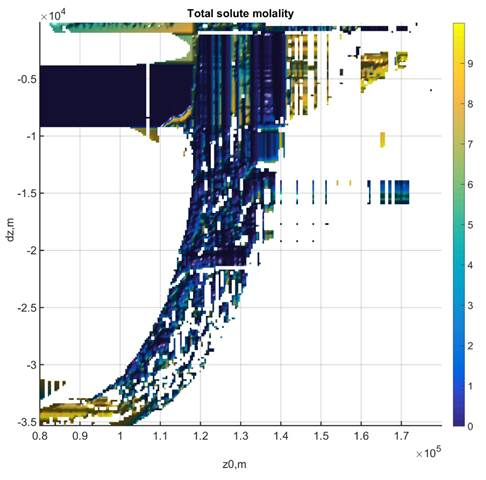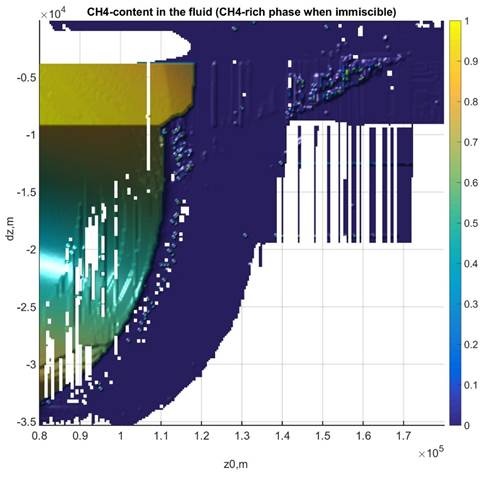Figure 4: Total solute molality and CH4- and CO2-content (mole fraction) in the fluid phase. Where no data is plotted the fluid is not stable. The data for this figure was generated with WERAMI (mode 2), property choice 40, and plotted in MATLAB with the perple_x_simple_plot script. The plot of methane concentration gives a false impression of the importance of such fluids in this example, i.e., the amount of the methane-rich fluid is minor.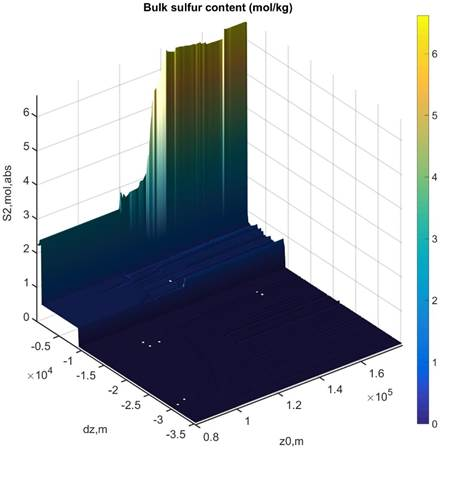Figure 5: Bulk sulfur content in the column. The pattern suggests that sulfur is scoured from the fluid as it infiltrates into progressively more silicic lithologies toward the slab surface. The three-dimensional perspective obscures the result that the maximum sulfur content is realized at the base of the sedimentary layer and that it subsequently decreases almost to the original sedimentary sulfur content at the slab interface. The data for this figure was generated with WERAMI (mode 2), property choice 6 (or 36) and plotted in MATLAB with the perple_x_simple_plot script.